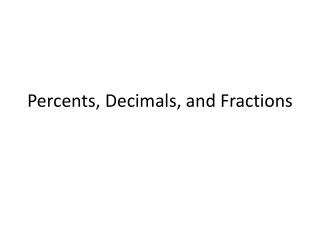DownloadDownload PresentationPercents, Decimals, and Fractions

# Percents, Decimals, and Fractions

Télécharger la présentation## Percents, Decimals, and Fractions

- - - - - - - - - - - - - - - - - - - - - - - - - - - E N D - - - - - - - - - - - - - - - - - - - - - - - - - - -
##### Presentation Transcript

1. Percents, Decimals, and Fractions

2. Percents • Means per hundred • 50% is 50 per hundred

3. Fractions numerator denominator

4. Converting from fractions to decimals to percents • Fraction to decimal • Divide the numerator by the denominator • Decimal to percent • Multiply by 100 (move the decimal point two places to the right) • Percent to decimal • Divide by 100 (move the decimal point two places to the left) • Decimal to fraction • Create a fraction using the place value

5. Example 1 • Write each number as a percent:

6. Example 2 • Write each number as a decimal and as a fraction:

7. Homework • P. 762 • 2-42 even